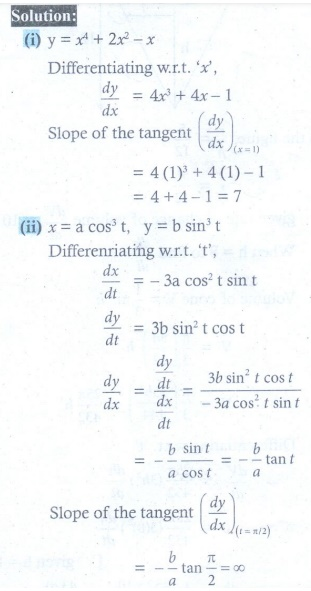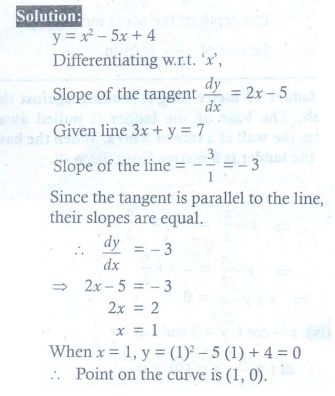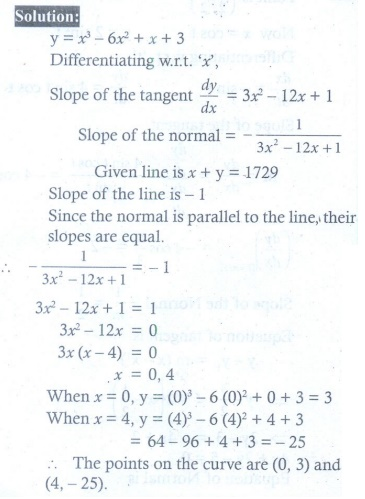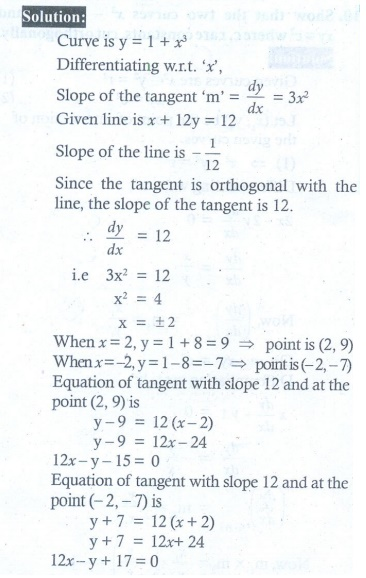Home | | Maths 12th Std | Exercise 7.2 : Equations of Tangent and Normal, Angle between two curves

# Exercise 7.2 : Equations of Tangent and Normal, Angle between two curves

Maths Book back answers and solution for Exercise questions - Mathematics : Applications of Differential Calculus : Equations of Tangent and Normal, Angle between two curves

EXERCISE 7.2

1. Find the slope of the tangent to the following curves at the respective given points.

(i) y = x4 + 2x2 ŌłÆ x at x = 1

(ii) = a cos3 t , y = b sin3 t at t = ŽĆ/2.2. Find the point on the curve y = x2 ŌłÆ 5x + 4 at which the tangent is parallel to the line 3x + y = 7 .3. Find the points on the curve = x3 ŌłÆ 6x2 + x + 3 where the normal is parallel to the line x + y = 1729 .4. Find the points on the curve y2 ŌłÆ 4xy = x2 + 5 for which the tangent is horizontal.5. Find the tangent and normal to the following curves at the given points on the curve.

(i) y = x2 ŌĆō x4 at (1, 0)

(ii) y = x4 + 2ex  at ( 0, 2)6. Find the equations of the tangents to the curve y = 1+ x3 for which the tangent is orthogonal with the line x + 12 y = 12 .7. Find the equations of the tangents to the curve y x+1 / x-1 which are parallel to the line x + 2 y = 6 .8. Find the equation of tangent and normal to the curve given by x = 7 cos t and y = 2sin tt Ōłł ŌäØ at any point on the curve.9. Find the angle between the rectangular hyperbola xy = 2 and the parabola x2 + 4y = 0.10. Show that the two curves x2 ŌłÆ y2 = r2 and xy = c2 where c , r are constants, cut orthogonally.(1) (i) 7 (ii) Ōł×

(2) (1, 0)

(3) ( 0, 3 and ( 4, ŌłÆ 25 )

(4) ( 2, ŌłÆ1) and (ŌłÆ2,1 )

(5) (i) 2x + y = 2 ; x ŌłÆ 2 y = 1 (ii) 2x ŌłÆ y = ŌłÆ2 ; x + 2 y = 4  (iii) x ŌłÆ y = 0 ; x + y = ŽĆ (iv) 4x + 2 y = 5 ; 2x ŌłÆ 4 y = ŌłÆ5

(6) 12x ŌłÆ y = 15 ;12x ŌłÆ y = ŌłÆ17

(7) x + 2 y = 7 ; x + 2 y = ŌłÆ1

(8) (2 cos t ) x + (7 sin t ) y = 14 ; (7 sin t ) x ŌłÆ (2 cos t ) y = 45sin t cos t

(9) tanŌłÆ1 (3)

Tags : Problem Questions with Answer, Solution , 12th Maths : UNIT 7 : Applications of Differential Calculus
Study Material, Lecturing Notes, Assignment, Reference, Wiki description explanation, brief detail
12th Maths : UNIT 7 : Applications of Differential Calculus : Exercise 7.2 : Equations of Tangent and Normal, Angle between two curves | Problem Questions with Answer, Solution